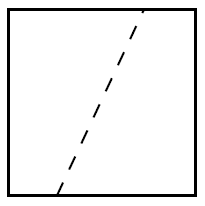#### You may also like### Square Areas

Can you work out the area of the inner square and give an explanation of how you did it?### Dissect

What is the minimum number of squares a 13 by 13 square can be dissected into?### 2001 Spatial Oddity

With one cut a piece of card 16 cm by 9 cm can be made into two pieces which can be rearranged to form a square 12 cm by 12 cm. Explain how this can be done.

# Square Bisection

##### Age 11 to 14 Short Challenge Level:

Any line which passes though the centre of the square divides the square into two congruent shapes. An example is shown below.There are an infinite number of suitable lines (lines passing through the centre, at any angle) so there are infinitely many ways the square can be cut in half with a single straight cut.
This problem is taken from the UKMT Mathematical Challenges.
You can find more short problems, arranged by curriculum topic, in our short problems collection.